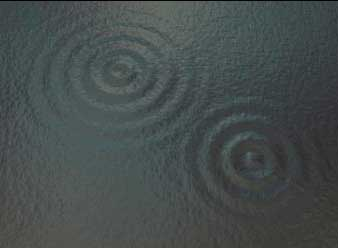If we think of a wave spreading out from a rock that is thrown into a pond, the further from the source, the bigger the circle formed by the wave. As the circle gets bigger, its total length (circumference) also gets bigger.Photo courtesy of Robert Simmon, NASA GSFC

Spreading loss occurs because the total amount of energy in a wave remains the same as it spreads out from a source. (We are neglecting sound absorption for the moment.) When the circle of a surface wave gets bigger the energy spreads to fill it. Therefore, the energy per unit length of the wave must get smaller. The height of the surface wave (amplitude) decreases as the energy per unit length of the wave crest gets smaller.

You can see something similar to spreading loss when you blow bubbles with chewing gum. Have you ever watched the bubble as it grows bigger? How does it change? Just as the total amount of energy in a sound wave doesn’t change as it spreads out, the total amount of chewing gum doesn’t change as the bubble gets bigger. This means that as the bubble grows, the walls of the bubble must get thinner and thinner. The thickness of the gum is similar to the amplitude of the sound wave. Just as the gum gets thinner as the bubble gets bigger, the amplitude of the sound wave decreases as it spreads out.

As surface waves spread out on the surface of a body of water, such as a pond or ocean surface, the amplitude gets smaller rapidly. This is called cylindrical spreading. Waves that spread out in all directions from a sound source, such as one in the middle of the ocean, get smaller even more rapidly than surface waves spreading out horizontally on a pond or ocean surface. This is called spherical spreading.

The following table compares the relative intensity and amplitude of sound waves at one meter from the source to their values at greater distances for cylindrical and spherical spreading.

Distance from Source Relative Intensity Relative Amplitude